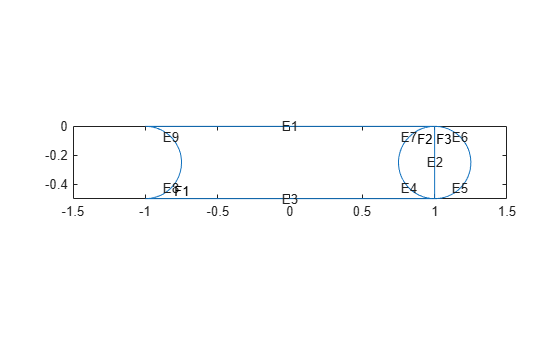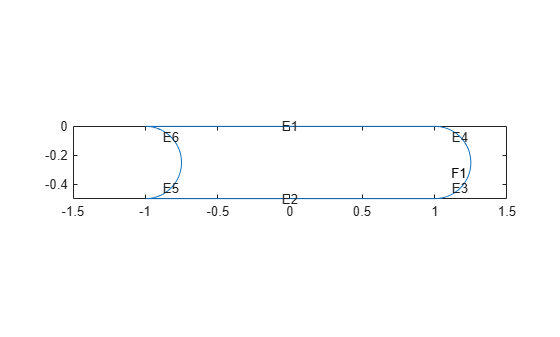## 2-D Geometry Creation at Command Line

### Three Elements of Geometry

To describe your geometry through Constructive Solid Geometry (CSG) modeling, use three data structures.

1. A matrix whose columns describe the basic shapes. When you export geometry from the PDE Modeler app, this matrix has the default name `gd` (geometry description).

2. A matrix whose columns contain names for the basic shapes. Pad the columns with zeros or 32 (blanks) so that every column has the same length.

3. A set of characters describing the unions, intersections, and set differences of the basic shapes that make the geometry.

### Basic Shapes

To create basic shapes at the command line, create a matrix whose columns each describe a basic shape. If necessary, add extra zeros to some columns so that all columns have the same length. Write each column using the following encoding.

Circle

RowValue
`1``1` (indicates a circle)
`2`x-coordinate of circle center
`3`y-coordinate of circle center
`4`Radius (strictly positive)

Polygon

RowValue
`1``2` (indicates a polygon)
`2`Number of line segments `n`
`3` through `3+n-1`x-coordinate of edge starting points
`3+n` through `2*n+2`y-coordinate of edge starting points

Note

Your polygon must not contain any self-intersections.

Rectangle

RowValue
`1``3` (indicates a rectangle)
`2``4` (number of line segments)
`3` through `6`x-coordinate of edge starting points
`7` through `10`y-coordinate of edge starting points

The encoding of a rectangle is the same as that of a polygon, except that the first row is `3` instead of `2`.

Ellipse

RowValue
`1``4` (indicates an ellipse)
`2`x-coordinate of ellipse center
`3`y-coordinate of ellipse center
`4`First semiaxis length (strictly positive)
`5`Second semiaxis length (strictly positive)
`6`Angle in radians from x axis to first semiaxis

### Rectangle with Circular End Cap and Another Circular Excision

Specify a matrix that has a rectangle with a circular end cap and another circular excision.

Create Basic Shapes

First, create a rectangle and two adjoining circles.

```rect1 = [3 4 -1 1 1 -1 0 0 -0.5 -0.5]; C1 = [1 1 -0.25 0.25]; C2 = [1 -1 -0.25 0.25];```

Append extra zeros to the circles so they have the same number of rows as the rectangle.

```C1 = [C1;zeros(length(rect1) - length(C1),1)]; C2 = [C2;zeros(length(rect1) - length(C2),1)];```

Combine the shapes into one matrix.

`gd = [rect1,C1,C2];`

Create Names for the Basic Shapes

In order to create a formula describing the unions and intersections of basic shapes, you need a name for each basic shape. Give the names as a matrix whose columns contain the names of the corresponding columns in the basic shape matrix. Pad the columns with 0 or 32 if necessary so that each has the same length.

One easy way to create the names is by specifying a character array whose rows contain the names, and then taking the transpose. Use the `char` function to create the array. This function pads the rows as needed so all have the same length. Continuing the example, give names for the three shapes.

```ns = char('rect1','C1','C2'); ns = ns';```

Set Formula

Obtain the final geometry by writing a set of characters that describes the unions and intersections of basic shapes. Use `+` for union, `*` for intersection, `-` for set difference, and parentheses for grouping. `+` and `*` have the same grouping precedence. `-` has higher grouping precedence.

Continuing the example, specify the union of the rectangle and `C1`, and subtract `C2`.

`sf = '(rect1+C1)-C2';`

Create Geometry and Remove Face Boundaries

After you have created the basic shapes, given them names, and specified a set formula, create the geometry using `decsg`. Often, you also remove some or all of the resulting face boundaries. Completing the example, combine the basic shapes using the set formula.

`[dl,bt] = decsg(gd,sf,ns);`

View the geometry with and without boundary removal.

```pdegplot(dl,'EdgeLabels','on','FaceLabels','on') xlim([-1.5,1.5]) axis equal```Remove the face boundaries.

```[dl2,bt2] = csgdel(dl,bt); figure pdegplot(dl2,'EdgeLabels','on','FaceLabels','on') xlim([-1.5,1.5]) axis equal```### Decomposed Geometry Data Structure

A decomposed geometry matrix has the following encoding. Each column of the matrix corresponds to one boundary segment. Any 0 entry means no encoding is necessary for this row. So, for example, if only line segments appear in the matrix, then the matrix has 7 rows. But if there is also a circular segment, then the matrix has 10 rows. The extra three rows of the line columns are filled with 0.

RowCircleLineEllipse
`1``1``2``4`
`2`Starting x coordinateStarting x coordinateStarting x coordinate
`3`Ending x coordinateEnding x coordinateEnding x coordinate
`4`Starting y coordinateStarting y coordinateStarting y coordinate
`5`Ending y coordinateEnding y coordinateEnding y coordinate
`6`Region label to left of segment, with direction induced by start and end points (`0` is exterior label)Region label to left of segment, with direction induced by start and end points (`0` is exterior label)Region label to left of segment, with direction induced by start and end points (`0` is exterior label)
`7`Region label to right of segment, with direction induced by start and end points (`0` is exterior label)Region label to right of segment, with direction induced by start and end points (`0` is exterior label)Region label to right of segment, with direction induced by start and end points (`0` is exterior label)
`8`x coordinate of circle center0x coordinate of ellipse center
`9`y coordinate of circle center0y coordinate of ellipse center
`10`Radius0Length of first semiaxis
`11`00Length of second semiaxis
`12`00Angle in radians between x axis and first semiaxis

## SupportGet trial now